# Machine Learning¶

Machine Learning deals with developing automatic algorithms for identifying patterns in complex data and for making prediction based on previous observations. Applications include search engines, image recognition, speech recognition, natural language processing, and much more. Machine Learning algorithms typically fit in either the supervised or unsupervised paradigm.

# Supervised Learning¶

In Supervised Learning, algorithms are trained on data labeled with a desired output. Here the machine (i.e., the algorithm) is expected to derive structure from the data using the presence of labels. A typical application of Supervised Learning is classification, i.e., the task of classifying a new test data point into one of the possible categories that are present in the training data. Image classification is the task of assigning a label to an image from a gives set of categories. For instance, given as input an image of a handwritten digit from 0 to 9, a classification algorithm should identify which category this data belongs to, i.e., which digit the image represents, out of the ten possible choices. Let's see what classification entails!

## Classifying points¶

While Python has many built-in datatypes and functions, typically applications in scientific computing and data science rely on datatypes and functions from external libraries (also called modules in Python). numpy is the core library for scientific computing, and it provides a high-performance array datatype (together with functions on them) that we will use repeadetly. matplotlib is a plotting library to plot numpy datatypes.

The following code imports these Python modules.

In :
import numpy as np
import matplotlib.pyplot as plt
# Configure matplotlib to embed the plots in the output cells of the present notebook
%matplotlib notebook


Assume we are given a collection of labeled two-dimensional points such as the one that we define below. Each point in X_train is labeled by a corresponding color in Y_train, either red or blue. The collections X_train and Y_train represent our training dataset.

In :
X_train = np.array([[1,1], [2,2.5], [3,1.2], [5.5,6.3], [6,9], [7,6]]) # Define numpy array of two-dim points
Y_train = ['red', 'red', 'red', 'blue', 'blue', 'blue'] # Define a Python built-in list (i.e., array) of strings


We can think of X_train as a two dimensional array. Notice that Python is a 0-indexed programming language, much like C!

In :
print(X_train[5,0]) # Extract the 0-th coordinate of the 5-th point in the array
print(X_train[5,1]) # Extract the 1-st coordinate of the 5-th point in the array

7.0
6.0


Python has a slicing syntax that allows us to extract multiple elements in an array at once. See the following code for instance.

In :
print(X_train[:,0]) # Extract all elements in the 1-st coordinate (indexed by 0) of the array X_train

[ 1.   2.   3.   5.5  6.   7. ]

In :
print(X_train[:,1]) # Extract all elements in the 2-st coordinate (indexed by 1) of the array X_train

[ 1.   2.5  1.2  6.3  9.   6. ]


The following lines of code use the slicing syntax to conviently plot this colletion of points, and their color label.

In :
plt.figure() # Define a new figure
plt.scatter(X_train[:,0], X_train[:,1], s = 170, color = Y_train[:]) # Plot points with Python slicing syntax
plt.show() # Display plot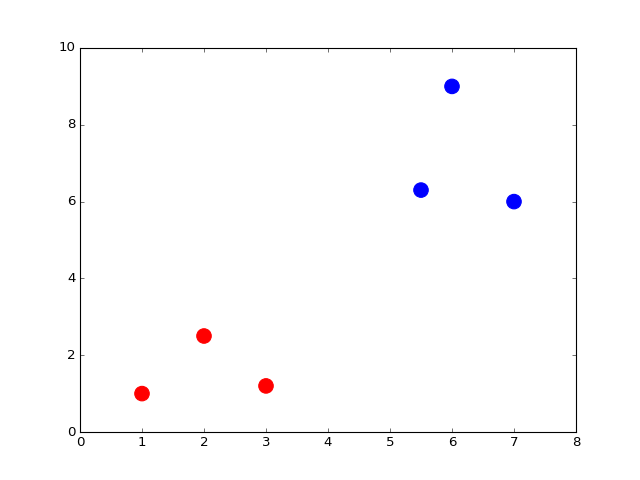Now, assume we are given a new test point X_test.

In :
X_test = np.array([3,4])


Let us see where this new point lands in the plot.

In :
plt.figure() # Define a new figure
plt.scatter(X_train[:,0], X_train[:,1], s = 170, color = Y_train[:])
plt.scatter(X_test, X_test, s = 170, color = 'green')
plt.show() # Display plot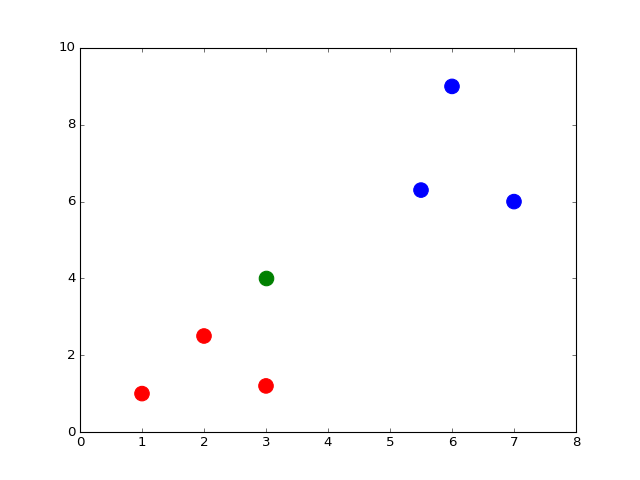Say we want to classify X_test as a being red or blue. One reasonable way to do so is to assign to X_test the same color of the point in X_train that is closest to X_test (its nearest neighbor). The following blocks of code precisely do this! First, we define a function that takes two generic points x and y and returns their Euclidean distance.

In :
def dist(x, y):
return np.sqrt(np.sum((x - y)**2)) # np.sqrt and np.sum are numpy functions to work with numpy arrays


Then, for each point in X_train we compute its distance from X_test, and we store this distance in the corresponding component of the array distance.

In :
num = len(X_train) # Compute the number of points in X_train
distance = np.zeros(num) # Initialize a numpy arrays of zeros
for i in range(num):
distance[i] = dist(X_train[i], X_test) # Compute distance from X_train[i] to X_test
print(distance)

[ 3.60555128  1.80277564  2.8         3.39705755  5.83095189  4.47213595]


As an aside, using the so-called vectorization syntax of Python we can write the output of the previous two blocks of code in only one line! In fact, this would be a preferred way of writing code, as for loops tend to be quite slow in Python (as compared to for loops in C). Just to spark up your interest in learning more about Python...

In :
distance = np.sqrt(np.sum((X_train - X_test)**2, axis = 1)) # Vectorization syntax
print(distance)

[ 3.60555128  1.80277564  2.8         3.39705755  5.83095189  4.47213595]


Second, we choose the point in X_train with the minimal distance from X_new, and we look at the color of it.

In :
min_index = np.argmin(distance) # Get the index with smallest distance
print(Y_train[min_index])

red


Per our specification, we should assign to X_test the color red!

Congratulations! What we have just implemented is the so-called Nearest Neighbor Classifier, our first Machine Learning algorithm! The Nearest Neighbor Classifier --- using precisely the same lines of code just described --- can be used to classify other type of datasets, such as the handritten digits dataset, as we see next.

## Classifying images¶

sklearn is a Python modules for machine learning. This module comes with a few standard datasets, such as the digits dataset. The following code loads this dataset from sklearn.

In :
from sklearn import datasets
digits = datasets.load_digits()


The digits dataset contains 1797 images representing handwritten digits, together with numerical labels representing the true number associated with each image. digits.images is the array of images, while digits.target is the array of labels. Python is a 0-indexed language, such as C, so these arrays are indexed from 0 to 1796.

Each element in the array digits.images is on its own a 8 by 8 array of pixels, where each pixel is an integer between 0 and 16. Let us look what the first image, indexed by 0, looks like:

In :
print(digits.images)

[[  0.   0.   5.  13.   9.   1.   0.   0.]
[  0.   0.  13.  15.  10.  15.   5.   0.]
[  0.   3.  15.   2.   0.  11.   8.   0.]
[  0.   4.  12.   0.   0.   8.   8.   0.]
[  0.   5.   8.   0.   0.   9.   8.   0.]
[  0.   4.  11.   0.   1.  12.   7.   0.]
[  0.   2.  14.   5.  10.  12.   0.   0.]
[  0.   0.   6.  13.  10.   0.   0.   0.]]


Can you see that the above represents number zero? It is easier if we plot this array by assigning to each number an intensity of black.

In :
plt.figure()
plt.imshow(digits.images, cmap = plt.cm.gray_r, interpolation = 'nearest')
plt.show()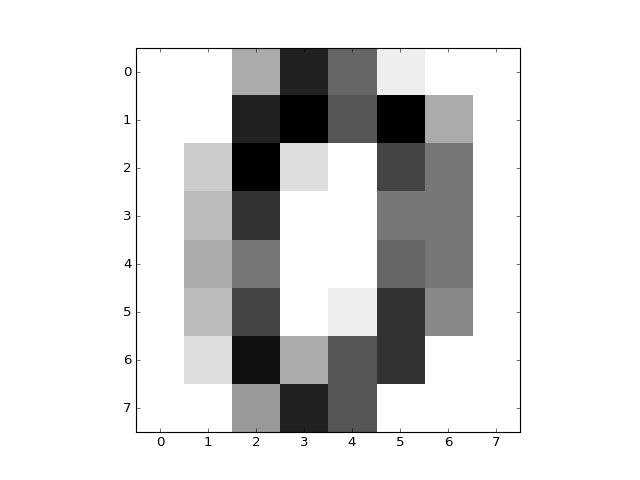Each element in the array digits.target is a numerical label representing the digit associated to the respective image.

In :
print(digits.target)

0


To evaluate the performance of an algorithm in Machine Learning, a common practice is to form subsets of the original dataset. One subset is the training set on which the data properties (i.e., the hidden structure) are learnt. Another subset is the testing set on which we test the performance of the algorithm. Here, let us form the training set by selecting all the labeled images from 0 to 9 (note that the slicing syntax [i:j] in Python means that we consider elements from i to j-1; so, in the following code the index 10 is not counted).

In :
X_train = digits.data[0:10]
Y_train = digits.target[0:10]


Suppose we now want to run the Nearest Neighbor Classifier described above to classify the following test data point (neglecting the fact that for this test data point we also have the true label available!)

In :
X_test = digits.data


We can see what this test point looks like.

In :
plt.figure()
plt.imshow(digits.images, cmap = plt.cm.gray_r, interpolation = 'nearest')
plt.show()We now run the Nearest Neighbor Classifier using exactly the same code written above to classify two dimensional points. In particular, note that we use the same function dist that was previously defined!

In :
num = len(X_train) # Compute the number of points in X_train
distance = np.zeros(num) # Initialize an arrays of zeros
for i in range(num):
distance[i] = dist(X_train[i], X_test) # Compute distance from X_train[i] to X_test
min_index = np.argmin(distance) # Get the index with smallest distance
print(Y_train[min_index])

3


Per our specification, we should assign to X_test the label 3. We can see that this does indeed corresponds to the true solution!

In :
print(digits.target[min_index])

3


So at least for the test point we tried, the algorithm worked well! To have a better idea of how well this algorithm does, we can repeat the above procedure to each element in the test dataset, which we consider being the set containing picture no. 1697 to no. 1796. The following code counts how many errors the algorithm commits over the test set. Note that the function range(i,j) creates a list of integers from i included to j-1, much like the slicing syntax we saw earlier.

In :
num = len(X_train)
no_errors = 0
distance = np.zeros(num)
for j in range(1697, 1797):
X_test = digits.data[j]
for i in range(num):
distance[i] = dist(X_train[i], X_test) # Compute distance from X_train[i] to X_test
min_index = np.argmin(distance)
if Y_train[min_index] != digits.target[j]:
no_errors += 1
print(no_errors)

37


So we have incurred in 37 errors out of 100 images in the test dataset! Not bad for a simple algorithm. But we can do much better if we enlarge the traning set, as we now see.

### Improving the performance¶

Much like humans get better at learning something the more they are exposed to it, also Machine Learning algorithms improve their performance with the amount of training data they are exposed to. This is the reason why much of the Machine Learning applications we use get better with time, the more we use them! Just think of speech recognition...

We can immediately observe this phenomenon in the case of the Nearest Neighbor Classifier, noticing that if we enlarge the amount of training data available to the algorithm, then the performance of the algorithm increases! For instance, let us see what happens if, instead of training the classifier with a set of 10 images (from 0 to 9), we train the classifier with a set of 1000 images (from 0 to 999).

In :
X_train = digits.data[0:1000]
Y_train = digits.target[0:1000]


We can now run the same code as above to count how many errors the algorithm commits over the same test set previously used.

In :
num = len(X_train)
no_errors = 0
distance = np.zeros(num)
for j in range(1697, 1797):
X_test = digits.data[j]
for i in range(num):
distance[i] = dist(X_train[i], X_test) # Compute distance from X_train[i] to X_test
min_index = np.argmin(distance)
if Y_train[min_index] != digits.target[j]:
no_errors += 1
print(no_errors)

3


Much better! As expected, now we have committed only 3 errors out of 100 images tried in the test set.

# Unsupervised Learning¶

In Unsupervised Learning, algorithms are trained with unlabeled data. Here the machine (i.e., the algorithm) is expected to derive structure from the data on its own, without the presence of labels that can guide the process as in Supervised Learning. A typical application of Unsupervised Learning is clustering, i.e., the task of grouping the data into k categories, where k can be given be the user or inferred directly by the algorithm. Let's see what clustering entails!

## Clustering points¶

First, we import the main Python modules we need for what follows.

In :
import numpy as np
import matplotlib.pyplot as plt
# To configure matplotlib to embed the plots in the output cells of the present notebook
%matplotlib notebook


Assume we are given a collection of (unlabeled!) two-dimensional points such as the one here defined and plotted.

In :
X = np.array([[1,1], [2,2.5], [3,1.2], [5.5,6.3], [6,9], [7,6], [8,8]]) # Define numpy array of two-dim points
plt.figure()
plt.scatter(X[:,0], X[:,1], s = 170, color = 'black') # Plot points with slicing syntax X[:,0] and X[:,1]
plt.show()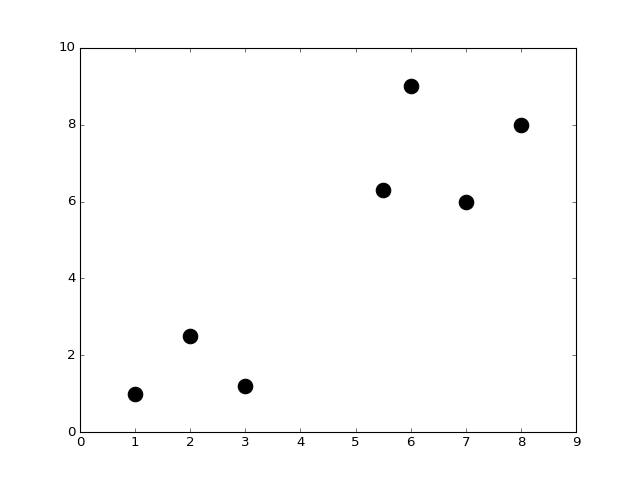Let us stress once again that this collection of points is unlabelled, that is, if you wish, each point now does not come with a pre-assigned color! (recall what was the case in Supervised Learning). Our job now is to cluster these points, that is, to assign colors to all of them!

Let's say that we want to cluster this collection of points into 2 groups, based on their vicinity. To the human eye this is an easy tak. What about computers? A popular Machine Lerning algorithm to do clustering is k-means. While the details of the k-means algorithm are not at all that difficult (also its implementation is not difficult), a proper exposition of k-means goes beyond the purpose of this class. As with SVM above, we now show how k-means can be easily imported and run in Python!

In :
from sklearn.cluster import KMeans


k-means divides the data points into k clusters. We begin with k=2.

In :
k = 2 # Define the number of clusters in which we want to partion the data
kmeans = KMeans(n_clusters = k) # Run the algorithm kmeans
kmeans.fit(X);
centroids = kmeans.cluster_centers_ # Get centroid's coordinates for each cluster
labels = kmeans.labels_ # Get labels assigned to each data


Let us now plot the points with label assignments as given by kmeans.

In :
colors = ['r.', 'g.'] # Define two colors for the plot below
plt.figure()
for i in range(len(X)):
plt.plot(X[i,0], X[i,1], colors[labels[i]], markersize = 30)
plt.scatter(centroids[:,0],centroids[:,1], marker = "x", s = 300, linewidths = 5) # Plot centroids
plt.show()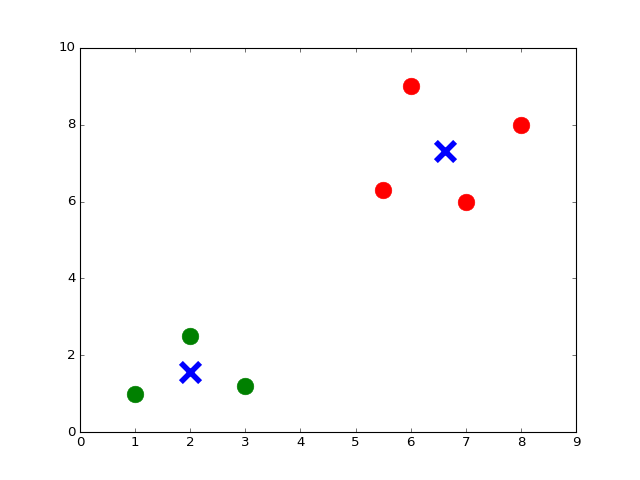The algorithm k-means divides the data into as many clusters as we want! Let us repeat the procedure above with k=3, group the code together.

In :
k = 3
kmeans = KMeans(n_clusters = k)
kmeans.fit(X);
centroids = kmeans.cluster_centers_
labels = kmeans.labels_
colors = ['r.', 'g.', 'y.']
plt.figure()
for i in range(len(X)):
plt.plot(X[i,0], X[i,1], colors[labels[i]], markersize = 30)
plt.scatter(centroids[:,0],centroids[:,1], marker = "x", s = 300, linewidths = 5)
plt.show()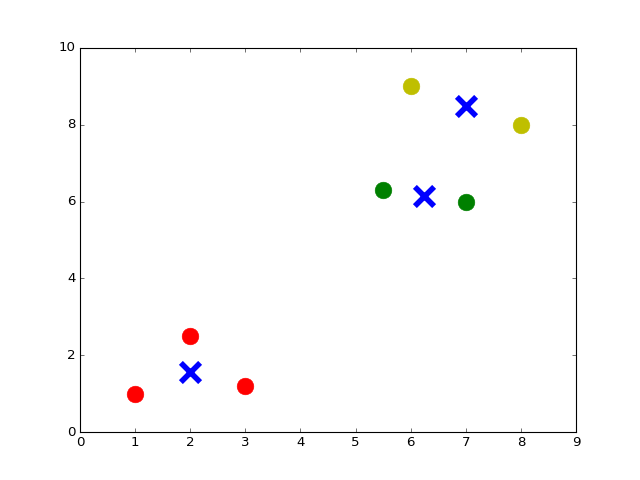Clearly, with k=7 we have the following.

In :
k = 7
kmeans = KMeans(n_clusters = k)
kmeans.fit(X);
centroids = kmeans.cluster_centers_
labels = kmeans.labels_
colors = ['r.', 'g.', 'y.', 'c.', 'b.', 'k.', 'm.']
plt.figure()
for i in range(len(X)):
plt.plot(X[i,0], X[i,1], colors[labels[i]], markersize = 30)
plt.scatter(centroids[:,0],centroids[:,1], marker = "x", s = 300, linewidths = 5)
plt.show()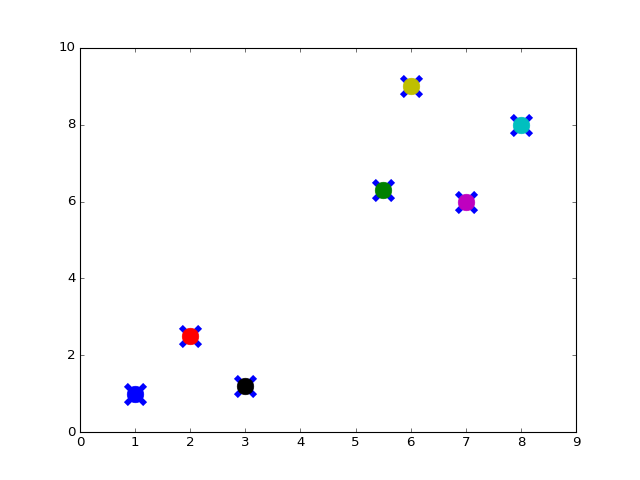The fact that k-means divides the data into as many clusters as we want is prototypical of many applications in Machine Learning. It is typically the case that users have some high-level control on the structure to be inferred. For instance, in this example it was evident that k=2 was a good choice! In other applications we may have other type of domain-specific insights that can guide the output of the algorithm. Of course, there are also algorithms that try to figure out the best k on their owns.. another time!

## Clustering documents¶

Ok, we know how to cluster points! What about documents? Suppose I am given a list of documents and I want to cluster these documents by their topic. Let us consider a quick example. Suppose we have the following corpus of documents, where each document is here represented by a string.

In :
corpus = ['I love CS50. Staff is awesome, awesome, awesome!',
'I have a dog and a cat.',
'Best of CS50? Staff. And cakes. Ok, CS50 staff.',
'My dog keeps chasing my cat. Dogs!'] # This represents a list of strings in Python


This set represents the data we want to cluster based on their topic. To the human eye it is clear that the documents can be grouped into two clusters: one group is about CS50, and it is made by document 0 and document 2; the other group is about dogs and cats, and it contains document 1 and document 3. Can we use the k-means algorithm to this end?

To run the k-means algorithm we need to transform this collection of documents into data that can be interpreted by the algorithm. That is, we need to transform each document into a numerical vector (i.e., a point in a certain high-dimensional space). The most intuitive way to do so is by using the bags of words representation, where each document is represented by its word count. First, we build a vocabulary from the words used in the corpus, when we do not consider the so-called "stop words" such as "a," "the," or "in," as they do not convey meaningful information. Then, for each document i in the collection, we count the number of occurrences of each word w in the vocabulary and we store it in Z[i,j], where j is the index of the word w in the vocabulary. The matrix Z represents the bags-of-words matrix, where each row represents a document in the corpus and each column represents a word in the vocabulary.

In :
# Create bags-of-words matrix
from sklearn.feature_extraction.text import CountVectorizer
count_vect = CountVectorizer(stop_words = 'english')
Z = count_vect.fit_transform(corpus)
# The function fit_transform() takes as input a list of strings and does two things:
# first, it "fits the model," i.e., it builds the vocabulary; second, it transforms the data into a matrix.


We can take a look at the words in the vocabulary.

In :
vocab = count_vect.get_feature_names()
print(vocab)

['awesome', 'best', 'cakes', 'cat', 'chasing', 'cs50', 'dog', 'dogs', 'keeps', 'love', 'ok', 'staff']


And we can look at the bags-of-words matrix Z.

In :
Z.todense() # Generate a dense matrix from Z, which is stored as a sparse matrix data-type

Out:
matrix([[3, 0, 0, 0, 0, 1, 0, 0, 0, 1, 0, 1],
[0, 0, 0, 1, 0, 0, 1, 0, 0, 0, 0, 0],
[0, 1, 1, 0, 0, 2, 0, 0, 0, 0, 1, 2],
[0, 0, 0, 1, 1, 0, 1, 1, 1, 0, 0, 0]], dtype=int64)

The bags-of-words matrix is a good start to associate a numerical value to each document, but also the lenght of each document should be taken into account. In fact, longer documents will tend to have higher absolute count values than shorter ones, even though they might talk about the same topics! To avoid these potential discrepancies we can divide the number of occurrences of each word in a document by the total number of words in the document, so that we get a frequency matrix. Another refinement is to have downscale weights for words that occur in many documents in the corpus, as these words tend to be less informative than those that occur only in a smaller portion of the corpus. The final weighted frequency matrix, which we call X, is tipically called tf–idf for “Term Frequency times Inverse Document Frequency”.

In :
# Create tf–idf matrix
from sklearn.feature_extraction.text import TfidfVectorizer
vectorizer = TfidfVectorizer(stop_words = 'english')
X = vectorizer.fit_transform(corpus)


And we can look at the tf–idf matrix.

In :
X.todense()

Out:
matrix([[ 0.89469821,  0.        ,  0.        ,  0.        ,  0.        ,
0.23513012,  0.        ,  0.        ,  0.        ,  0.29823274,
0.        ,  0.23513012],
[ 0.        ,  0.        ,  0.        ,  0.70710678,  0.        ,
0.        ,  0.70710678,  0.        ,  0.        ,  0.        ,
0.        ,  0.        ],
[ 0.        ,  0.35415727,  0.35415727,  0.        ,  0.        ,
0.55844332,  0.        ,  0.        ,  0.        ,  0.        ,
0.35415727,  0.55844332],
[ 0.        ,  0.        ,  0.        ,  0.38274272,  0.48546061,
0.        ,  0.38274272,  0.48546061,  0.48546061,  0.        ,
0.        ,  0.        ]])

Now we have a good numerical representation of our corpus. In order to run k-means on this representation it is convenient to choose a different notion of distance between points. We can now run the k-means algorithm to divide the data points into k=2 clusters.

In :
k = 2 # Define the number of clusters in which we want to partion THE data
# Define the proper notion of distance to deal with documents
from sklearn.metrics.pairwise import cosine_similarity
dist = 1 - cosine_similarity(X)
# Run the algorithm KMeans
model = KMeans(n_clusters = k)
model.fit(X);


To visualize the outcome of the algorithm, we can print the top terms per cluster.

In :
print("Top terms per cluster:\n")
order_centroids = model.cluster_centers_.argsort()[:, ::-1]
terms = vectorizer.get_feature_names()
for i in range(k):
print ("Cluster %i:" % i, end='')
for ind in order_centroids[i, :3]:
print (' %s,' % terms[ind], end='')
print ("")

Top terms per cluster:

Cluster 0: awesome, staff, cs50,
Cluster 1: dog, cat, keeps,


## Example: clustering movies based on their IDMB synopses¶

Ok, now that we have an idea of how to cluster collection of documents, why not trying with something more interesting? After all, the example above is so small that the human eye is more than enough! Something more interesting is the following. This is a list of movies I remember watching at some point in my life https://docs.google.com/spreadsheets/d/1udJ4nd9EKlX_awB90JCbKaStuYh6aVjh1X6j8iBUXIU/, with their synopses taken from the IDMB website. We can easily import this table to Python with the followin code.

In :
# Import the data set into a Panda data frame
import pandas as pd
from io import StringIO
import requests

act = requests.get('https://docs.google.com/spreadsheets/d/1udJ4nd9EKlX_awB90JCbKaStuYh6aVjh1X6j8iBUXIU/export?format=csv')
dataact = act.content.decode('utf-8') # To convert to string for Stringio
frame = pd.read_csv(StringIO(dataact))


Take a look at this data frame to realize that it has the same content as the Google spreedsheet.

In :
print(frame)

                     Title                                           Synopsis
0       Mad Max: Fury Road  Max Rockatansky (Tom Hardy) explains in a voic...
1               The Matrix  The screen is filled with green, cascading cod...
2        The King's Speech  The film opens with Prince Albert, Duke of Yor...
3   No Country for Old Men  The film opens with a shot of desolate, wide-o...
4         A Beautiful Mind  John Nash (Russell Crowe) arrives at Princeton...
5                Inception  A young man, exhausted and delirious, washes u...
6                   Frozen  The Walt Disney Pictures logo and the movie ti...
7            The Lion King  The Lion King takes place in the Pride Lands o...
8                  Aladdin  The film starts with a street peddler, guiding...
9               Cinderella  The film opens in a tiny kingdom, and shows us...
10            Finding Nemo  Two clownfish, Marlin (Albert Brooks) and his ...
11               Toy Story  A boy called Andy Davis (voice: John Morris) u...
12              Robin Hood  Told with animals for it's cast, the story tel...


We are going to apply the k-means algorithm to this data, clustering according to the topic inferred by the movie synopsis. To do so, we need to select the Synopsis and convert them into a list of strings, such as the corpus of four documents given in the previous example.

In :
# Loop over each synopsis and append its content to a list of string named 'corpus'
corpus = []
for i in range(0, frame["Synopsis"].size):
corpus.append(frame["Synopsis"][i])


Then we create the tf–idf matrix as done previously, with the additional requirement that we consider only the words that appear in at least 20% of the documents. You can try to remove this requirement and see what happens!

In :
# Create tf–idf matrix
from sklearn.feature_extraction.text import TfidfVectorizer
vectorizer = TfidfVectorizer(stop_words = 'english', min_df = 0.2)
# min_df = 0.2 means that the term must be in at least 20% of the documents
X = vectorizer.fit_transform(corpus)


Now we are ready to run the k-means algorithm.

In :
k = 2 # Define the number of clusters in which we want to partion our data
# Define the proper notion of distance to deal with documents
from sklearn.metrics.pairwise import cosine_similarity
dist = 1 - cosine_similarity(X)
# Run the algorithm kmeans
model = KMeans(n_clusters = k)
model.fit(X);


To visualize the outcome of the algorithm, we can print the title of the movie in each cluster, plus the top words per cluster.

In :
no_words = 4 # Number of words to print per cluster
order_centroids = model.cluster_centers_.argsort()[:, ::-1] # Sort cluster centers by proximity to centroid
terms = vectorizer.get_feature_names()
labels = model.labels_ # Get labels assigned to each data

print("Top terms per cluster:\n")
for i in range(k):

print("Cluster %d movies:" % i, end='')
for title in frame["Title"][labels == i]:
print(' %s,' % title, end='')
print() #add a whitespace

print("Cluster %d words:" % i, end='')
for ind in order_centroids[i, :no_words]:
print (' %s' % terms[ind], end=','),
print()
print()

Top terms per cluster:

Cluster 0 movies: Mad Max: Fury Road, The Matrix, No Country for Old Men, A Beautiful Mind, Inception, Frozen, Finding Nemo, Toy Story,
Cluster 0 words: room, tank, says, joe,

Cluster 1 movies: The King's Speech, The Lion King, Aladdin, Cinderella, Robin Hood,
Cluster 1 words: king, prince, john, palace,



Ok, the algorithm figured out that my tastes split into the Walt Disney type and action movies ;)